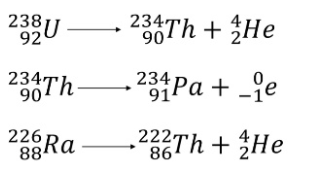The spontaneous emission of certain invisible radiations from the nuclei of some substances is called radioactivity.

The phenomenon of spontaneous emission of highly penetrating radiations from heavy substances(A> 206) occurring in the nature is called natural radioactivity.The phenomenon of converting light stable nuclei into unstable form by bombarding with it some energized particles(nuclear transmutation) is known as artificial radioactivity.deflection in electric field                                       deflection in magnetic field

In the above figure, a small amount of radioactive substance was taken in a lead block which continuously emitted certain radiations . The radiations that deflected towards the negative plate was alpha rays, that deflected toward positive plate was beta rays and that showed no deviation was gamma rays. Alpha and beta rays were deflected in magnetic field but gamma rays showed no deviation.

Alpha rays:

(a) It is a stream of alpha particles, each particle containing two protons and two neutrons. An alpha particle is nothing but a helium nucleus.

(b) Being made of positively charged particles, alpha ray can be deflected by an electric field as well as by a magnetic field.

(c) Its penetrating power is low. Even in air, its intensity falls down to very small values within a few centimetres.

(d) Alpha rays coming from radioactive materials travel at large speeds of the order of 10 6 m s –1.

(e)   All the alpha particles coming from a particular decay scheme have the same energy.

(f) Alpha ray produces scintillation (flashes of light) when it strikes certain fluorescent materials, such as barium platinocyanide.

(g) It causes ionization in gases.

Beta rays:

(a) It is a stream of electrons coming from the nuclei. Thus, the properties of beta ray, cathode ray, thermions, photoelectrons, etc., are all identical except for their origin. Beta particles are created at the time of nuclear transformation, whereas, in cathode ray, thermions, etc., the electrons are already present and get ejected.

(b) Being made of negatively charged particles, beta ray can be deflected by an electric field as well as by a magnetic field.

(c) Its penetrating power is greater than that of alpha ray. Typically, it can travel several metres in air before its intensity drops to small values.

(d) The ionizing power is less than that of alpha rays.

(e) Beta ray also produces scintillation in fluorescent materials, but the scintillation is weak.

(f) The energy of the beta particles coming from the same decay scheme are not equal. This is because the available energy is shared by antineutrinos. The energy of beta particles thus varies between zero and a maximum.

Gamma rays:

(a) Gamma-ray is electromagnetic radiation of a short wavelength. Its wavelength is, in general, smaller than X-rays. Many of its properties are the same as those of X-rays.

(b) Being chargeless, it is not deflected by electric or magnetic field.

(c) It has the least ionizing power and the largest penetrating power among different types of nuclear radiation.

(d) All the photons coming from a particular gamma decay scheme have the same energy.

(e) Being an electromagnetic wave, gamma ray travels in vacuum with the velocity c.

Alpha decay:

In alpha decay, the unstable nucleus emits an alpha particle reducing its proton number Z as well as its neutron number N by 2. The alpha decay process may be represented as.As the proton number Z is changed, the element itself is changed, and hence the chemical symbol of the residual nucleus is different from that of the original nucleus. The nucleus before the decay is called the parent nucleus and that resulting after the decay is called the daughter nucleus. An example of alpha decay isBeta decay:

A beta particle (Denoted by 𝛽) is a fast-moving electron which is emitted from the nucleus of an atom undergoing radioactive decay. Beta-decay occurs when a neutron changes into a proton and an electron.

As a result of beta decay, the nucleus has one less neutron, but one extra proton. The atomic number, Z, increases by 1, and the mass number, A, stays the same.Gamma decay:

Very often, a nucleus after undergoing radioactive decay is left in the excited state. Then nucleus undergoes a second decay by release excess energy in the form of photon and becomes stable. Such photons emitted are in fact gamma rays.